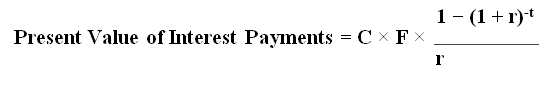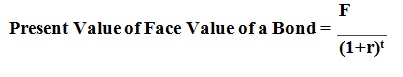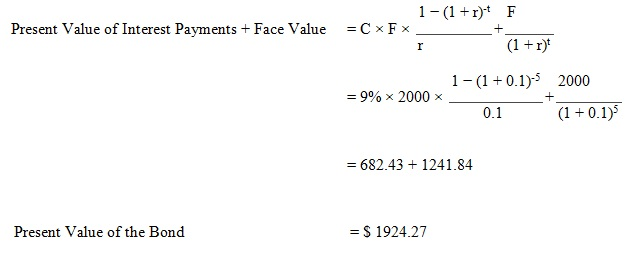# How to Calculate Present Value of a Bond

## What is a bond

A bond is a financial instrument that is issued for a specific period with the purpose of borrowing money. When the bond is issued, it promises the holder, to pay a fixed sum of interest based on the predefined interest rate (coupon rate) at specified dates, usually, semi-annually, annually,etc. until it matures and it repays the principle amount at the maturity.

## Present Value of a Bond

Present value is an alternative bond valuation method that calculates the current worth of the stream of future cash flows at a given rate of return. Cash flows on a bond are fairly certain. So, the present value of a bond is the value equal to the discounted interest payments (interest inflows) and the discounted redemption value of the face value of the bond certificate. These cash flows will be discounted based on the interest rate prevailing in the market at a particular instant.

## Calculate present value of a bond:

### Step 1: Calculate Present Value of the Interest Payments

Present value of the interest payments can be calculated using following formula where,

C = Coupon rate of the bond
F = Face value of the bond
R = Market
t = Number of time periods occurring until the maturity of the bond### Step 2: Calculate Present Value of the Face Value of the Bond

This refers to the maturity value of the bond, which can be calculated using the following formula.### Step 3: Calculate Present Value of Bond

The total present value of the bond can be represented as,## Calculate Present value of a bond – Example:

Following information is given with regard to the bond issue of ABC Company.

Face value of the bond – \$ 2000
Maturity period of the bond – 5 years
Annual coupon rate – 9%
Market interest rate – 10%

Assuming that ABC Company pays annual coupon payments, calculate the present value of the bond.Assuming that ABC Company pays its interest on semi-annual basis, calculate the present value of the bond.

Since the company pays the interest semi-annually, both coupon rate and market rate has to be adjusted per period. Therefore, there are 10 time periods to bond to be mature and coupon rate and interest rate will be 4.5% and 5% per period respectively.### Recap

A bond is a financial debt instrument. Calculating present value of a bond involves discounting coupon income based on the market interest rate plus discounting the face value of the bond after the maturity period. This value represents the current value of the future cash flows that will be generated by this instrument.